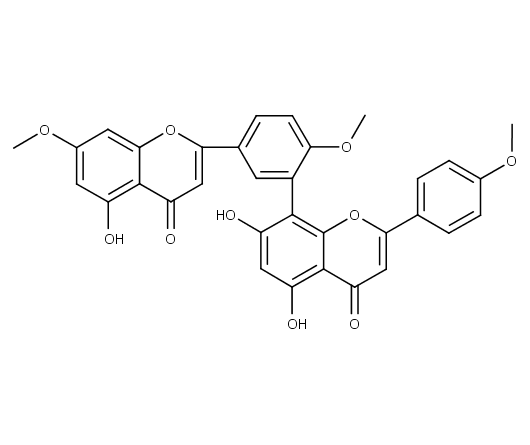Sciadopitysin analytical standard provided with w/w absolute assay, to be used for quantitative titration.
Synonym
• Amentoflavone-4',4''',7''-trimethylether
182.00 \$
182.00 \$

## identification

1021 S
C₃₃H₂₄O₁₀
521-34-6
580.54
Amentoflavone-4',4''',7''-trimethylether
COC1=CC=C(C=C1)C1=CC(=O)C2=C(O)C=C(O)C(=C2O1)C1=CC(=CC=...
COC1=CC=C(C=C1)C1=CC(=O)C2=C(O)C=C(O)C(=C2O1)C1=CC(=CC=C1OC)C1=CC(=O)C2=C(O)C=C(OC)C=C2O1

## specification

Sciadopitysin analytical standard provided with w/w absolute assay, to be used for quantitative titration.
Purity (HPLC) ≥99% (This product is available with HPLC chromatogram)

P262 P260

## storage

Store at : <+8°C
Store in dry and dark place.
Ambient temperature